# 回归分析及预测性能评估（通过python的scikit-learn实现）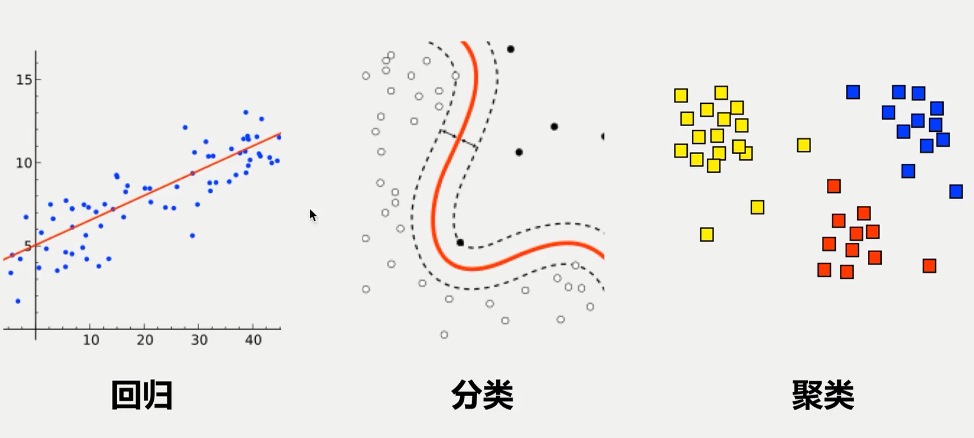#### 下面我们说说回归分析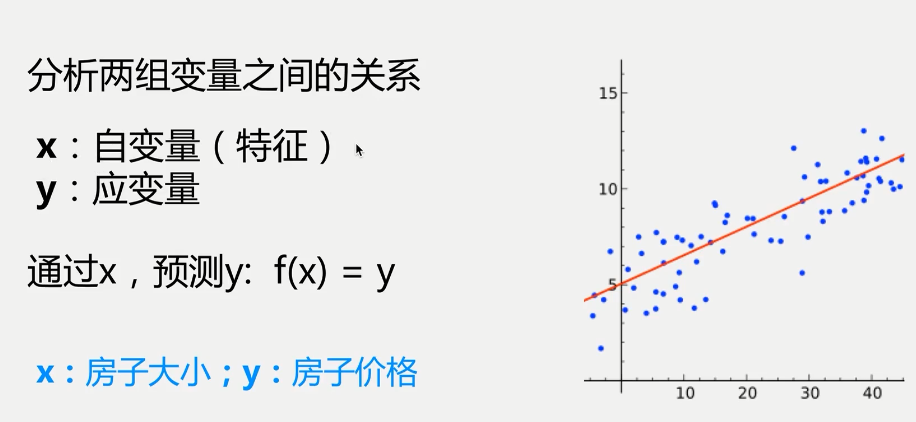x自变量也叫特征，可以是一维或者多维，y是因变量，我们通过已知的x和y，建立一个y和x之间的函数来评估x和y之间的关系，进行用新的x去预测y。

Python中实现线性回归的主流包是scikit-learn，下面我们一步一步来通过scikit-learn实现回归分析和预测性能的评估。

from sklearn import linear_model #引入python的sklearn模块
lm = linear_model.LinearRegression() #创建一个线性回归模型
model = lm.fit(X,y)#对回归模型进行拟合

#### 1 加载数据（鸢尾属植物数据集）

mport pandas
iris.columns=['SepalLengthCm','SepalWidthCm','PetalLengthCm','PetalWidthCm','Species']

 iris.sample(10)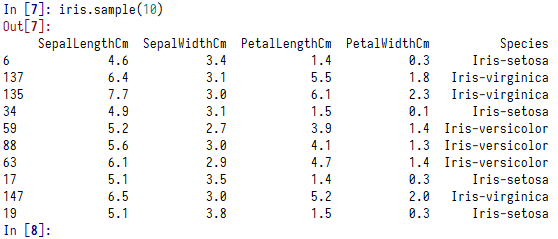• epalLengthCm：花萼长度
• SepalWidthCm：花萼宽度
• PetalLengthCm：花瓣长度
• PetalWidthCm：花瓣宽度
• Species：分类（ Iris-setosa、Iris-virginica、Iris-versicolour）

#### 2 探索数据

 iris.drop_duplicates(['Species'])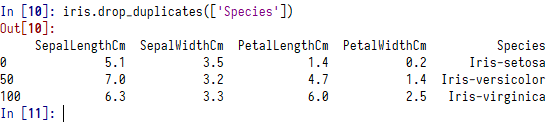import seaborn as sns
sns.regplot(x='PetalLengthCm',y='PetalWidthCm',data=iris)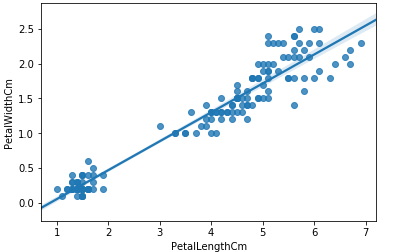#### 3 回归拟合

lm = linear_model.LinearRegression() #创建回归模型
features = ['PetalLengthCm'] #定义特征值，就是选项自变量，可以多个，多个的写法features = ['a','b']
X = iris[features] #从数据集中选取特征值数据
y = iris['PetalWidthCm'] #定义因变量
model = lm.fit(X,y) #y=a+bX进行拟合
print(model.intercept_,model.coef_) #输出截距a和特征系数b

-0.366514045217 [ 0.41641913]

y=-0.3665+0.4164X

model.predict(3.5)

Out: array([ 1.09095292])

lm = linear_model.LinearRegression()
features = ['PetalLengthCm','SepalLengthCm']
X = iris[features]
y = iris['PetalWidthCm']
model = lm.fit(X,y)
print(model.intercept_,model.coef_)

-0.013852011013 [ 0.44992999 -0.08190841]

 model.predict([[1,3]])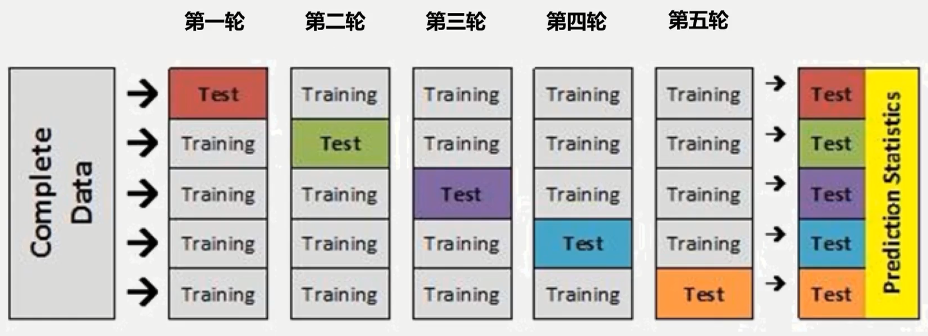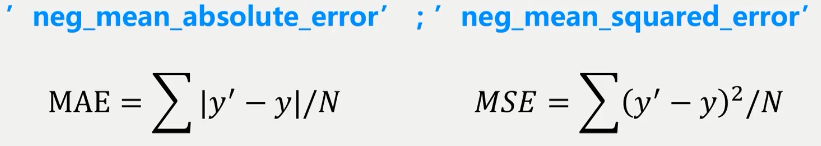from sklearn.model_selection import cross_val_score
cross_val_score(lm,X,y,cv=5,scoring='neg_mean_absolute_error')

lm就是我们上面建立的模型，cv=5是指样本的划分份数，你可以设置为10或者更大都可以，scoring就是我们的打分函数。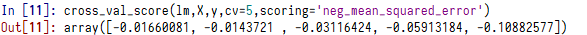• 需要注意的是在sklearn中cross_val_score的打分函数中返回的是MAE、MSE的负数，所以在cross_val_score中返回的MAE和MSE是负数，这个值越大性能越好。
• 但我还是看正数舒服一点，那么我们就在cross_val_score函数前面增加一个负号，这样cross_val_score得到的值就是和我们上面MAE和MSE公式的值是一直的，越小越好。
•  cv=5，进行了5轮评估，cross_val_score返回一个数组，分别是5轮的性能得分，我们可以计算它们的平均值作为最终的得分。

lm = linear_model.LinearRegression()
features = ['PetalLengthCm']
X = iris[features]
y = iris['PetalWidthCm']
model = lm.fit(X,y)
score = numpy.mean(-cross_val_score(lm,X,y,cv=5,scoring='neg_mean_squared_error'))
print(score)

socre结果为：0.0454809790262

score = numpy.mean(-cross_val_score(lm,X,y,cv=10,scoring='neg_mean_squared_error'))


socre结果为：0.047206999550184515

lm = linear_model.LinearRegression()
features = ['PetalLengthCm','SepalLengthCm']
X = iris[features]
y = iris['PetalWidthCm']
model = lm.fit(X,y)
score = numpy.mean(-cross_val_score(lm,X,y,cv=5,scoring='neg_mean_squared_error'))
print(score)

socre结果为：0.0460189525758

lm = linear_model.LinearRegression()
features = ['PetalLengthCm','SepalLengthCm','SepalWidthCm']
X = iris[features]
y = iris['PetalWidthCm']
model = lm.fit(X,y)
score = numpy.mean(-cross_val_score(lm,X,y,cv=5,scoring='neg_mean_squared_error'))
print(score)

socre结果为：0.0395036603861

def combinations(ls):
n=1<<len(ls)
tmp=[]
for i in range(n):
bits=[i>>offset&1 for offset in range(len(ls)-1,-1,-1)]
if numpy.sum(bits)>0:
current=[ls[index] for (index,bit) in enumerate(bits) if bit==1]
#print(current);
#print([tmp,current])
tmp= tmp+[current]
return tmp

group=['PetalLengthCm', 'SepalLengthCm', 'SepalWidthCm']
group_combinations = combinations(group)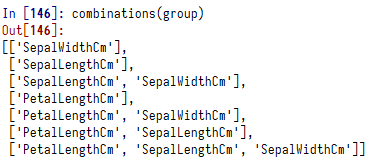cv=5 #定义样本分组的大小
for v in group_combinations:
X = iris[v]
y = iris['PetalWidthCm']
model = lm.fit(X,y)
score = numpy.mean(-cross_val_score(lm,X,y,cv=cv,scoring='neg_mean_squared_error'))
print('特征'+str(v) +'的平均得分：'+ '%.4f' % score)

• 特征[‘SepalWidthCm’]的平均得分：0.7661
• 特征[‘SepalLengthCm’]的平均得分：0.2436
• 特征[‘SepalLengthCm’, ‘SepalWidthCm’]的平均得分：0.1917
• 特征[‘PetalLengthCm’]的平均得分：0.0455
• 特征[‘PetalLengthCm’, ‘SepalWidthCm’]的平均得分：0.0443
• 特征[‘PetalLengthCm’, ‘SepalLengthCm’]的平均得分：0.0460
• 特征[‘PetalLengthCm’, ‘SepalLengthCm’, ‘SepalWidthCm’]的平均得分：0.0395

model.predict([[5,6,3]])
model.score(X,y)

from sklearn import linear_model
import pandas
import seaborn as sns
from sklearn.model_selection import cross_val_score
import numpy
from itertools import combinations

iris.columns=['SepalLengthCm','SepalWidthCm','PetalLengthCm','PetalWidthCm','Species']

sns.regplot(x='PetalLengthCm',y='PetalWidthCm',data=iris)

lm = linear_model.LinearRegression()
features = ['PetalLengthCm']
X = iris[features]
y = iris['PetalWidthCm']
model = lm.fit(X,y)
score = numpy.mean(-cross_val_score(lm,X,y,cv=5,scoring='neg_mean_squared_error'))
print('平均性能得分：'+str(score))
print('截距:'+str(model.intercept_),'特征系数：',model.coef_)

lm = linear_model.LinearRegression()
features = ['PetalLengthCm','SepalLengthCm']
X = iris[features]
y = iris['PetalWidthCm']
model = lm.fit(X,y)
score = numpy.mean(-cross_val_score(lm,X,y,cv=5,scoring='neg_mean_squared_error'))
print('平均性能得分：'+str(score))
print('截距:'+str(model.intercept_),'特征系数：',model.coef_)

lm = linear_model.LinearRegression()
features = ['PetalLengthCm','SepalLengthCm','SepalWidthCm']
X = iris[features]
y = iris['PetalWidthCm']
model = lm.fit(X,y)
score = numpy.mean(-cross_val_score(lm,X,y,cv=5,scoring='neg_mean_squared_error'))
print('平均性能得分：'+str(score))
print('截距:'+str(model.intercept_),'特征系数：',model.coef_)

def combinations(ls):
n=1<<len(ls)
tmp=[]
for i in range(n):
bits=[i>>offset&1 for offset in range(len(ls)-1,-1,-1)]
if numpy.sum(bits)>0:
current=[ls[index] for (index,bit) in enumerate(bits) if bit==1]
tmp= tmp+[current]
return tmp

cv=5
group =  ['PetalLengthCm', 'SepalLengthCm', 'SepalWidthCm']
group_combinations = combinations(group)
for v in group_combinations:
X = iris[v]
y = iris['PetalWidthCm']
model = lm.fit(X,y)
score = numpy.mean(-cross_val_score(lm,X,y,cv=cv,scoring='neg_mean_squared_error'))
print('特征'+str(v) +'的平均得分：'+ '%.4f' % score)

#### 请关注我的微信公众号